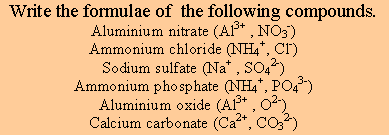Writing Ionic Formulae

When writing the formula of ionic compounds, it is best to refer to the chart of ions and their valency shown on the right.

 Charge of 1+ Charge of 2+ Charge of 3+ Ammonium(NH4+) Magnesium(Mg2+) Aluminium(Al3+) Hydrogen(H+) Calcium(Ca2+) Chromium(Cr3+) Lithium(Li+) Barium(Ba2+) Iron(III)(Fe3+) Sodium(Na+) Zinc(Zn2+) Potassium(K+) Copper(II)(Cu2+) Silver(Ag+) Mercury(Hg2+) Copper(Cu+) Iron(II)(Fe2+) Nickel(Ni2+) Tin(II)(Sn2+) Lead(II)(Pb2+) Charge of 1- Charge of 2- Charge of 3- Hydroxide(OH-) Oxide(O-2) Nitride(N-3) Hydrogen sulfide(HS-) Sulfide(S-2) Phosphate(PO4-3) Nitrite(NO2-) Sulfate(SO4-2) Nitrate(NO3-) Sulfite(SO3-2) Acetate(CH3COO-) Carbonate(CO3-2) Fluoride(F-) Dichromate(Cr2O7-2) Chloride(Cl-) Bromide(Br-) Iodide(I-) Permanganate(MnO4-)
When writing the formula of an ionic compound a few steps must be followed.
Step 1 - Write the formula of each ion and its charge.
Step 2 - Balance the positive and the negative charges.
Step 3 - Use subscripts to write the formula.
Step 4 - Use brackets if the ion has more than one capital letter and more than one ion is required.
Example 1 What is the formula of aluminium sulfate.
Step 1 - Al+3 and SO4-2
Step 2 - Balance the positive and negative charges.
In order to balance 3+ and 2- we have to find the lowest common multiple.
In this case it is 6.
In order to get 6+ we need 2 Aluminium ions.
In order to get 6- we need 3 sulfate ions.
Step 3 and 4 Write the formula using subscript.
Al2(SO4)3

Note how we used brackets around the sulfate ion. That's because sulfate is an ion with two capital letters and we need more than one sulfate ion.

If you prefer to be more visual, try this

What is the formula for aluminium oxide?
Step 1 - Write the formula of each ion and its charge.
Step 2 - Balance the positive and the negative charges.
Step 3 - Use subscripts to write the formula.
Step 4 - Use brackets if the ion has more than one capital letter and more than one ion is required.

What is the formula for lead phosphate?
Step 1 - Write the formula of each ion and its charge.
Step 2 - Balance the positive and the negative charges.
Step 3 - Use subscripts to write the formula.
Step 4 - Use brackets if the ion has more than one capital letter and more than one ion is required.Example 2 Write the formula of copper sulfate
Step 1 Cu+2, SO4-2
Step 2 Balance the positive and negative charges.
Copper has a charge of 2+ and sulfate has a charge of 2-.
They are balanced.
We need one of each.
Step 3 Write the formula using subscript.
CuSO4
Note. No brackets were needed because we only need ONE sulfate ion.
No numbers (subscripts) were needed because we have one of each ion.

Click to see a good exercise to help you write ionic formulae

Write the formula for the compound formed between calcium and carbon known as calcium carbide. Write the formula for aluminium sulfate and ammonium phosphate

View the video on right for the solution( *only for senior chemistry students) Click to see how the valency of an ion can be used to generate the formula of a compound

More exercises